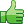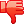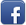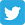# How to sum these output values

Posted on 16th Feb 2014 07:03 pm by admin

Hi

I need sum the month totals
SELECT region_name, area_name ,SUM (CASE WHEN SUBSTR(yr_mth,5,2) = '07' THEN sales_target END) AS jul ,SUM (CASE WHEN SUBSTR(yr_mth,5,2) = '08' THEN sales_target END) AS aug ,SUM (CASE WHEN SUBSTR(yr_mth,5,2) = '09' THEN sales_target END) AS sep ,SUM (CASE WHEN SUBSTR(yr_mth,5,2) = '10' THEN sales_target END) AS oct ,SUM (CASE WHEN SUBSTR(yr_mth,5,2) = '11' THEN sales_target END) AS nov ,SUM (CASE WHEN SUBSTR(yr_mth,5,2) = '12' THEN sales_target END) AS dec ,SUM (CASE WHEN SUBSTR(yr_mth,5,2) = '01' THEN sales_target END) AS jan ,SUM (CASE WHEN SUBSTR(yr_mth,5,2) = '02' THEN sales_target END) AS feb ,SUM (CASE WHEN SUBSTR(yr_mth,5,2) = '03' THEN sales_target END) AS mar ,SUM (CASE WHEN SUBSTR(yr_mth,5,2) = '04' THEN sales_target END) AS apr ,SUM (CASE WHEN SUBSTR(yr_mth,5,2) = '05' THEN sales_target END) AS may ,SUM (CASE WHEN SUBSTR(yr_mth,5,2) = '06' THEN sales_target END) AS jun FROM sales_forecast WHERE REGION_NAME like nvl('®IONNAME','%') AND AREA_NAME like nvl('&AREANAME','%') AND yr_mth=nvl('&Year',substr(yr_mth,1,4))||NVL(DECODE('&Month','JAN','01','FEB','02','MAR','03','APR','04','MAY','05', 'JUN','06','JUL','07','AUG','08','SEP','09','NOV','11','DEC','12'),substr(yr_mth,5,6)) GROUP BY region_name, area_name ORDER BY region_name, area_name Requirment region_name, area_name, jul, aug ................ jun abc xyz 10, 20.....................30 ccc ggg 20, 30.....................40 total 30, 50 ...................70
thanks

24523##### Other forums

how do i display data on a page from mysql
1 - do i use this code at the top of each of my page i wish to only alow access if there as been a s

Using Windows message as a Handle
Hi,

I am writing one of my first multithreaded programs.

Click counter to ignore traffic from search bots
I have a click counter on my site that...well, counts the number of clicks a link gets on the frontp

Remove directories not empty
hi,
i have an array that contains some names (1,4,6,56...). Every integer is a name of a folder.<

php/xmlrpc class issue